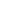# Free CBSE Class 10 Mathematics UNIT VI: Mensuration Areas related to circles Worksheets

Download free printable Areas related to circles Worksheets to practice. With thousands of questions available, you can generate as many Areas related to circles Worksheets as you want.

## Sample CBSE Class 10 Mathematics UNIT VI: Mensuration Areas related to circles Worksheet Questions

1.

what is the area of base of cone?

1.

2πr

2.

πr

3.

πr2

4.

3πr

2.

Find the area of minor segment whose chord substends and radius of circle is 42 cm.

3.

The perimeter of a sector of a circle of radius 5.7 m is 27.2 m. Calculate area of the sector.

4.

If the perimeter of a circle is equal to that of a square, then the ratio of their area is

5.

A pendulum swings through on angle of and describes an arc 6.6 cm in length. Find the length of pendulum.

6.

The length of the minute hand of a clock is 7 cm. Find the area swept by the minute hand in 10 minutes.

7.

A square is inscribed in a circular table cloth of radius 35 cm. If the cloth is to be coloured leaving the square . Find the area to be coloured.

1. 700 sq cm 2. 1400 sq cm 3. 2100 sq cm 4. 2800 sq cm
8.

If the circumference of a circle and the perimeter of a square are equal, then ________.

1. Area of the circle = Area of the square 2. Area of the circle < Area of the square 3. Area of the circle > Area of the square 4. None of these
9.

Area of the largest triangle that can be inscribed in a semi-circle of radius r units is ________.

1. 2. 3. 4.
10.

The cost of fencing a circular field at the rate of Rs 24 per metre is Rs 5280. The field is to be ploughed at the rate of Rs 1 per . Find the cost of ploughing the field.

1. Rs 3850 2. Rs 1925 3. Rs 7700 4. None of these

Worksheets by UrbanPro

Our worksheets are designed to help students explore various topics, practice skills and enrich their subject knowledge, to improve their academic performance. Designed by Experts who have extensive experience and expertise in teaching a subject, these worksheets will improve your child's problem-solving skills and subject knowledge in a fun and interactive manner.
Check out our free customized worksheets across school boards, grades, subjects and levels of subject knowledge. You can download, print and share these worksheets with anyone, anywhere, anytime!

Get a custom worksheet to practice!

Select your topic & see the magic.

subjectSelect Chapter(s)

Chapters & Subtopics